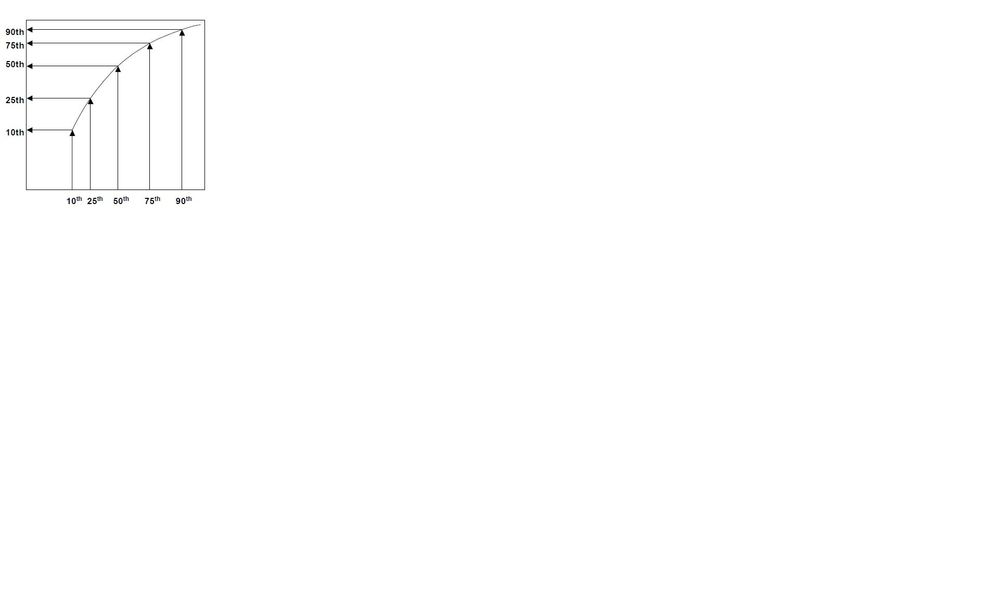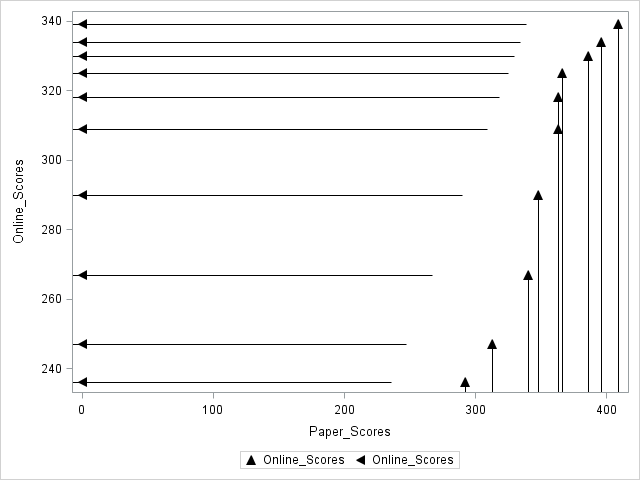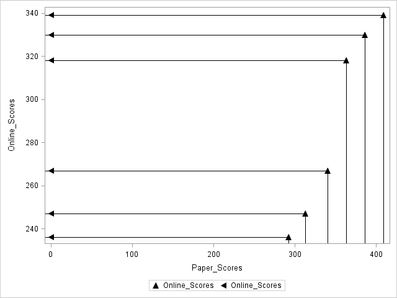## matching percentiles on the graph

Hi,

I'd like to create a graph as below. I have a data set including two variables. x axis will be one of the variables' percentiles and y axis will have the other one's percentiles. I did something in excel but I couldn't draw the arrows. Is there a way to draw that fancy graph?

Thanks1 ACCEPTED SOLUTION

Accepted Solutions

## Re: matching percentiles on the graph

This gets you a bit closer, but not all the way there. For the final 'finish' you can create the labels needed for your graph using a format to map your values to a specific label. Not sure why it's not working here but it's pretty straightforward.

``````*create sample data;
data demo;
do i=1 to 100;
paper_scores = rand('normal', 40, 5);
online_scores = rand('normal', 60, 8);
output;
end;
run;

*get percentiles for graph;
ods select none;
proc means data=demo min p10 p20 p30 p40 p50 p60 p70 p80 p90 max stackods;
var paper_scores online_scores;
ods output summary = ss;
run;
ods select all;

*transpose to a different structure to support graphing;
proc transpose data=ss out=ss_long;
id variable;
run;

data ss_long;
set ss_long;
*clean up statistics names;
name = substr(_name_, 1, length(_name_) - 4);

*rename values;
if _label_ = 'Maximum' then percentile = 100;
else if _label_ = 'Minimum' then percentile = 0;
else percentile = input(compress(_label_, , 'kd'), 8.);

*calculate arrow start and end for each plot;

*You probably don't need this step but it just makes the next steps easier;
arrow_up_start_x = paper_scores;
arrow_up_start_y = 0;
arrow_up_end_x = paper_scores;
arrow_up_end_y = online_scores;

arrow_left_start_x = paper_scores;
arrow_left_start_y = online_scores;
arrow_left_end_x = 0;
arrow_left_end_y = online_scores;
run;

proc sort data=ss_long;
by percentile name;
run;

*create formats to customize labels;
data x_format;
set ss_long;
start = paper_scores;
label = percentile;
type = 'N';
fmtname = 'xaxis_fmt';
run;

data y_format;
set ss_long;
start = online_scores;
label = percentile;
type = 'N';
fmtname = 'yaxis_fmt';
run;

proc format cntlin=x_Format;
run;

proc format cntlin=y_Format;
run;

*create graph;
proc sgplot data=ss_long noautolegend;
spline x=paper_scores y = online_scores;
vector x=arrow_up_end_x y=arrow_up_end_y / xorigin = arrow_up_start_x yorigin = arrow_up_start_y;
vector x=arrow_left_end_x y=arrow_left_end_y / xorigin = arrow_left_start_x yorigin = arrow_left_start_y;

xaxis label = 'Paper Scores'  valuesformat = xaxis_fmt. min=0;
yaxis label = 'Online Scores' valuesformat = yaxis_fmt.  min=0;
run;

``````
11 REPLIES 11PGStats
Opal | Level 21

## Re: matching percentiles on the graph

If you wanted just the lines, you could use DROPLINE in SGPLOT. For arrows, you will need VECTOR in SGPLOT. for the curve, use SPLINE.

PG

## Re: matching percentiles on the graph

Or via GTL, using DRAWARROW statements.Ksharp
Super User

## Re: matching percentiles on the graph

``````data x;
set sashelp.class(firstobs=16);
x1=0;
run;

proc sgplot data=x;
scatter x=weight y=height/ markerattrs=(symbol=trianglefilled size=10 color=black);
dropline x=weight y=height/ dropto=x lineattrs=(color=black);

scatter x=x1 y=height/ markerattrs=(symbol=triangleleftfilled size=10 color=black);
dropline x=weight y=height/ dropto=y lineattrs=(color=black);
run;

``````

## Re: matching percentiles on the graph

Thank you @Ksharp ,

This code works but this is based on data. I'd like to draw the graph with percentiles (P5 P10 P20 P30 P40 P50). Is it possible to do that?

## Re: matching percentiles on the graph

Hi @Ksharp again,

I calculated percentiles in excel and applied your code on the new file. Here is the graph. Do you think there is a way to connect the arrows. There is some space between arrows?

ThanksKsharp
Super User

## Re: matching percentiles on the graph

Or try VECTOR .

Or try HIGHLOW .

``````proc sgplot data=x;series  x=weight y=height ;
scatter x=weight y=height/ markerattrs=(symbol=trianglefilled size=10 color=black);
dropline x=weight y=height/ dropto=x lineattrs=(color=black);

scatter x=x1 y=height/ markerattrs=(symbol=triangleleftfilled size=10 color=black);
dropline x=weight y=height/ dropto=y lineattrs=(color=black);
run;``````

## Re: matching percentiles on the graph

Thank you so much @Ksharp . Here is how the new graph looks.. The arrows look good now, the only thing is x and y values' labels. As seen in the first post the y and x values are '5th', '10th' etc. but in my graph I have the exact values. If there is no way to change, then I will just provide this graph.Ksharp
Super User

## Re: matching percentiles on the graph

Using option 'values' of xaxis. to display 5th instead of 100 .

``````proc sgplot data=x;
scatter x=weight y=height/ markerattrs=(symbol=trianglefilled size=10 color=black);
dropline x=weight y=height/ dropto=x lineattrs=(color=black);

scatter x=x1 y=height/ markerattrs=(symbol=triangleleftfilled size=10 color=black);
dropline x=weight y=height/ dropto=y lineattrs=(color=black);xaxis values=('5th' '10th' ............ ) ;
run;``````

## Re: matching percentiles on the graph

An example (without the arrows) is shown in the article "Fit a distribution from quantiles," which includes the SAS code to create it.

As mentioned by others, you can use the VECTOR statement if arrows are essential.

## Re: matching percentiles on the graph

This gets you a bit closer, but not all the way there. For the final 'finish' you can create the labels needed for your graph using a format to map your values to a specific label. Not sure why it's not working here but it's pretty straightforward.

``````*create sample data;
data demo;
do i=1 to 100;
paper_scores = rand('normal', 40, 5);
online_scores = rand('normal', 60, 8);
output;
end;
run;

*get percentiles for graph;
ods select none;
proc means data=demo min p10 p20 p30 p40 p50 p60 p70 p80 p90 max stackods;
var paper_scores online_scores;
ods output summary = ss;
run;
ods select all;

*transpose to a different structure to support graphing;
proc transpose data=ss out=ss_long;
id variable;
run;

data ss_long;
set ss_long;
*clean up statistics names;
name = substr(_name_, 1, length(_name_) - 4);

*rename values;
if _label_ = 'Maximum' then percentile = 100;
else if _label_ = 'Minimum' then percentile = 0;
else percentile = input(compress(_label_, , 'kd'), 8.);

*calculate arrow start and end for each plot;

*You probably don't need this step but it just makes the next steps easier;
arrow_up_start_x = paper_scores;
arrow_up_start_y = 0;
arrow_up_end_x = paper_scores;
arrow_up_end_y = online_scores;

arrow_left_start_x = paper_scores;
arrow_left_start_y = online_scores;
arrow_left_end_x = 0;
arrow_left_end_y = online_scores;
run;

proc sort data=ss_long;
by percentile name;
run;

*create formats to customize labels;
data x_format;
set ss_long;
start = paper_scores;
label = percentile;
type = 'N';
fmtname = 'xaxis_fmt';
run;

data y_format;
set ss_long;
start = online_scores;
label = percentile;
type = 'N';
fmtname = 'yaxis_fmt';
run;

proc format cntlin=x_Format;
run;

proc format cntlin=y_Format;
run;

*create graph;
proc sgplot data=ss_long noautolegend;
spline x=paper_scores y = online_scores;
vector x=arrow_up_end_x y=arrow_up_end_y / xorigin = arrow_up_start_x yorigin = arrow_up_start_y;
vector x=arrow_left_end_x y=arrow_left_end_y / xorigin = arrow_left_start_x yorigin = arrow_left_start_y;

xaxis label = 'Paper Scores'  valuesformat = xaxis_fmt. min=0;
yaxis label = 'Online Scores' valuesformat = yaxis_fmt.  min=0;
run;

``````

## Re: matching percentiles on the graph

Thank you so much @Reeza  and @Ksharp

Best,

Discussion stats
• 11 replies
• 2113 views
• 2 likes
• 5 in conversation